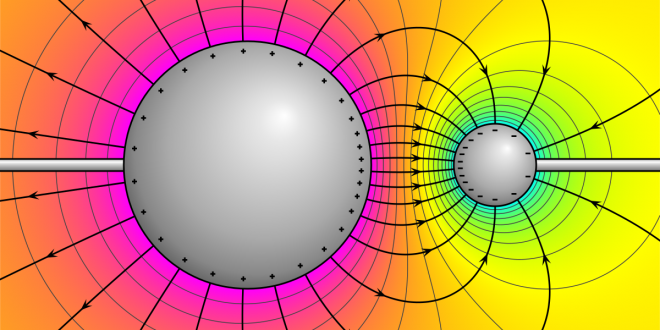Sunday , November 28 2021# Concept of Electric Field Due to Point Charge Revisited

The physics statement “Every charge in the universe exerts a force on every other charge in the universe” is bold but correct. Imagine the influence of the charge as a field to appreciate its potential better to influence other charges everywhere in space. It’s important to note that this ‘impact’ is simply the electrostatic force that a charge can apply to another charge.

In this article, we will look at the concept of electric field due to point charge derivation.

Coulomb’s Law

Coulomb’s Law is defined as the law that the electrostatic force exerted on a test object by another object is proportional to the test object’s charge rather than its mass. What do you get if you divide the Coulomb force by the charge of the test object, using the same logic as gravity?

The result is yet another quantity that is unrelated to the test object. This time, however, it is not due to the acceleration. The electric field (also known as the E-field) is entirely determined by the charge of the other object participating in the electrical contact.

The Concept of an Electric Field

It is important to know that a field is actually a way to think about and map the force surrounding anything that tends to act on another distant object, even when there is actually no physical relationship.

It is noteworthy that the gravitational field that tends to surround the planet earth and other masses, for example, that tends to depict the gravitational pull which is felt if another mass was placed on a specific point inside the field.

To make things easier, we’d like a field that depends on Q and does not on the test charge q so the electric field can be defined so that it only represents the charge that generates it, and it is unique at every location in space. The field that is electric E, in particular, is defined as the ratio of the force of Coulomb to the test charge:

E= F/q

Electrostatic Force

F. denotes the electrostatic force exerted on a positively charged q It is clear that E travels in the same direction as F. It’s also supposed that q is so little that it does not affect the electric field’s charge distribution. Newtons per Coulomb (N/C) are the units of field that is electric.

If you know the electric field, you can calculate the electrostatic force on any other charge q by multiplying the charge by the electric field, or F = qE. Take a look at the field that is electric produced by a point charge Q. Coulomb’s law states that the force exerted on a test charge q is

=The electrostatic force field that surrounds a charged object stretches outward in all directions.

The electrostatic force, which is given out by a point charge on your test charge at a distance r, is proportional to the charge given out on charges and if we talk about the amount of distance that is covered them.

E = F/q denotes the electric field, with F denoting the Coulomb or electrostatic force exerted on a minor positive test charge q. The letter E is made up of N/C units.

E = (k*Q)/r is called the magnitude is given out by the electric field E formed with the help of a point charge Q.

It’s worth noting that the electric field is a vector quantity that exists at every point in space and whose value is solely determined by the radial distance from q. The test charge q0 is capable of producing an electric field. As a result, we should make the test charge as modest as possible to avoid its influence.

Electric Field Due To Point Charge

Consider a point charge Q placed in a vacuum at the origin O. According to Coulomb’s law, if another point charge q is placed at a position P where OP = r, the charge Q will exert a force on q. The charge Q creates an electric field that may be felt all around the place.

The field at point P acts on a fresh charge, q, and generates a force. The electric field produced by a charge Q at r can be represented as follows:

A unit vector from the origin to the point r equals r = r/r. As a result, for each value of the position vector r, the above statement provides the electric field. The term “field” refers to the variation in the position of a distributed quantity (which could be a scalar or a vector).

The presence of an electric field has been paired with the action of a charge. The force F that a charge Q exerts on another charge q is computed as:

Conclusion

Now that you know all about the electric field concept because of a point charge keep reading to increase your knowledge base.

## Exam Hall Tips to do well in IMO in class 6th

No matter how well you prepare for an exam, your result is determined by the …